# OOF: Finite Element Analysis of Microstructures

cubicNext: hexagonal Up: Element Types and their Previous: empty   Contents

Subsections

## cubic

These are elements with cubic symmetry. According to figure 4.2 there are three independent entries in the stiffness matrix. We pick those independent coefficients with a somewhat standard convention for the cubic Young's modulus (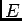), Poisson's ratio (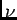) and the anisotropy factor (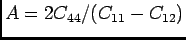) which makes the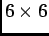matrix form of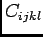look like: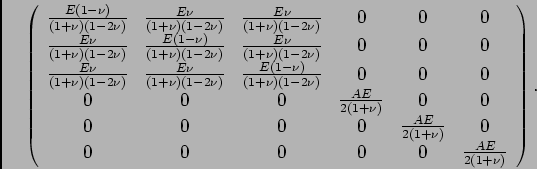This is equivalent to the isotropic stiffness matrix when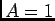.

The two-dimensional stiffness matrix depends on whether planestrain is true or false.

### Parameters

young
The cubic Young's modulus[stress].

poisson
The cubic Poisson's ratio[dimensionless].

anisotropy
The anisotropy factor,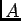[dimensionless].

alpha
The coefficient of thermal expansion. In cubic materials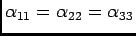; all off-diagonal terms vanish. [inverse temperature]

orientation
The orientation of the crystalline axes. See Section 4.3. [degrees]

/* Send mail to the OOF Team *//* Go to the OOF Home Page */